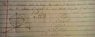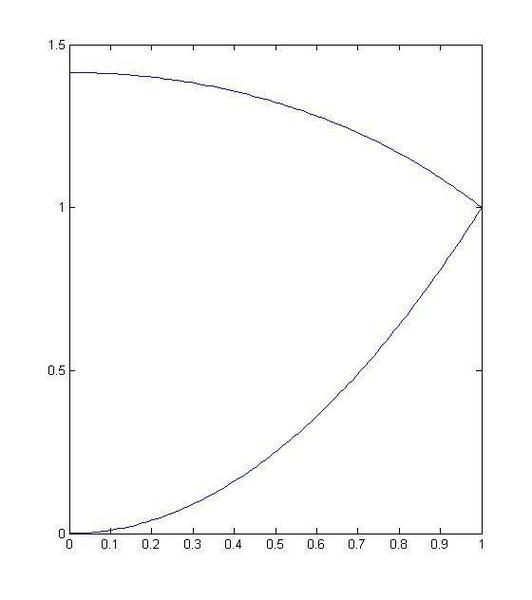# Finding centroid of a solid

• Phil Frehz

## Homework Statement

Use cylindrical coordinates to find the centroid of the solid.

The solid that is bounded above by the sphere x2 + y2 + z2 = 2

and below by z = x2 + y2

## Homework Equations

x = rcos(theta)

y= rsin(theta)[/B]

## The Attempt at a Solution

I am having trouble trying to find the limits of integration for r.

I have been able to get the sphere in terms of r as z = (2-r2)1/2 and the paraboloid as z = r2

I understand that in cylindrical coordinates the region is occupied from 0<theta<2pi and r originates from origin n to the uppermost part of the sphere.

I also found that when you equate both surface z equations. you get r2 = (2-r2)1/2 . Solving for r you get radical 2 and 1. r being the furthest distance would then have the upper limit of 1. I'm not sure if this is the correct method of solving.[/B]

#### Attachments

•IMG_20150722_125134.jpg
31.7 KB · Views: 458

First off, ## \sqrt 2>1## and ##\sqrt 2## doesn't seem to solve your equation.

Go with z=1 for the intersection point, so z is defined by the sphere above 1<z <2 and as the paraboloid for 0<z <1.

•Phil Frehz
See the attached image, this is the shape you are dealing with (revolved around the z axis to form a volume). Clearly, your centroid will be at x=0, y=0. How will you find the centroid in the z direction?•Phil Frehz
See the attached image, this is the shape you are dealing with (revolved around the z axis to form a volume). Clearly, your centroid will be at x=0, y=0. How will you find the centroid in the z direction?
View attachment 86271

So what I did was found out the value of z at the intersection point by equating both surfaces in terms of r, which led z to be either -2 or 1. When inputting z =1 i get r to also be 1

The centroid in the z direction will thus be 02pi 01 r2√(2-r2) z ⋅ r dz dr dθ all divided by the volume of the region

Last edited:
So what I did was found out the value of z at the intersection point by equating both surfaces in terms of r, which led z to be either -2 or 1. When inputting z =1 i get r to also be 1

Do you mean you solved ##r^2 = (2-r^2)^{1/2}## as a quadratic to find ## r^2 = 1 \text{ or } -2 ## and then discarded the imaginary solution?
The centroid in the z direction will thus be 02pi 01 r2√(2-r2) z ⋅ r dz dr dθ all divided by the volume of the region

Any troubles with these integrals?

Do you mean you solved ##r^2 = (2-r^2)^{1/2}## as a quadratic to find ## r^2 = 1 \text{ or } -2 ## and then discarded the imaginary solution?

Any troubles with these integrals?

Yes I discarded the imaginary solution, I have no problem solving the integrals. I only had trouble identifying the upper limit of r. Would you say that the way I solved for r was a correct solution?

That is the correct way to do it.
What you did wrong on your original work posted in the image was to go from
## r^4 + r^2 =2 ## to r =1 or r = sqrt(2); you should have gotten r =1 or r = sqrt(-2).

•Phil Frehz
That is the correct way to do it.
What you did wrong on your original work posted in the image was to go from
## r^4 + r^2 =2 ## to r =1 or r = sqrt(2); you should have gotten r =1 or r = sqrt(-2).

Ah I overlooked the proper solution for r. Thanks for taking the time to help me out!